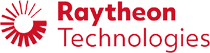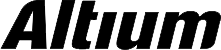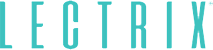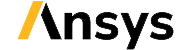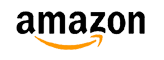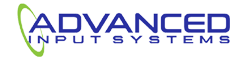﻿ Modeling High Frequency PCBs as Causal Systems | NWES Blog## Modeling High Frequency PCBs as Causal Systems

By ZM Peterson • Dec 18, 2019

Advanced PCBs are pushing signal frequencies and digital signal bandwidths to their limits, and designers need to make themselves aware of all the tools available to properly design their boards for high performance. Not all PCB modeling and simulation tools give designers access to accurate time dependent models for describing interconnect behavior.

Signal and power integrity problems like ringing, crosstalk, and jitter are well-known in systems that run at sub-ns switching speeds, regardless of data rates. In high frequency/high speed PCB design, correctly modeling all of these effects (and many more) is critical for electrical and structural design. Some models for describing interconnect behavior produce simulation results that are quite different from measurements. In some cases, this is due to problems with treating PCB interconnects as causal systems.

Creating models for causal systems is not as difficult as it sounds, and folks in the signal processing community are likely familiar with this process. In this post, I’ll introduce a brief guide that explains how to create a causal model for PCBs. These models are very important for modeling signal behavior and power behavior in a real PCB, especially when you are working in the GHz range.

## What are Causal Systems and Causal Models?

A causal systems model is one where the response in a system to some stimulus only appears some time after the system excited. As an example, consider a transmission line being driven with a digital signal. The digital signal and any ringing it produces will only appear on the transmission line after the driver’s state switches.

If this sounds obvious, then you are thinking about signal behavior correctly. Of course a signal should not propagate on an interconnect until after the driver switches! The problem with simple PCB models is that they predict signal behavior that is non-causal. Going back to the example of a transmission line, the signal appears on an interconnect before the driver switches. Obviously, this behavior does not match what we observe in reality.

When going back to signal behavior, noncausal models tend to produce poor predictions of signal behavior in a PCB above ~4 GHz, both in transmission lines and in power delivery networks. The complex relationships between power integrity, signal integrity, stackup design, conducted and radiated EMI, and impedance control requires careful modeling of any PCB as a causal system. Incorrect signal and power behavior models can leave you trying to diagnose potential signal and power integrity problems that merely appear as artifacts. Let’s take a look at the foundation of causal systems, their models, and how to properly build causal models for signal and power behavior in PCBs.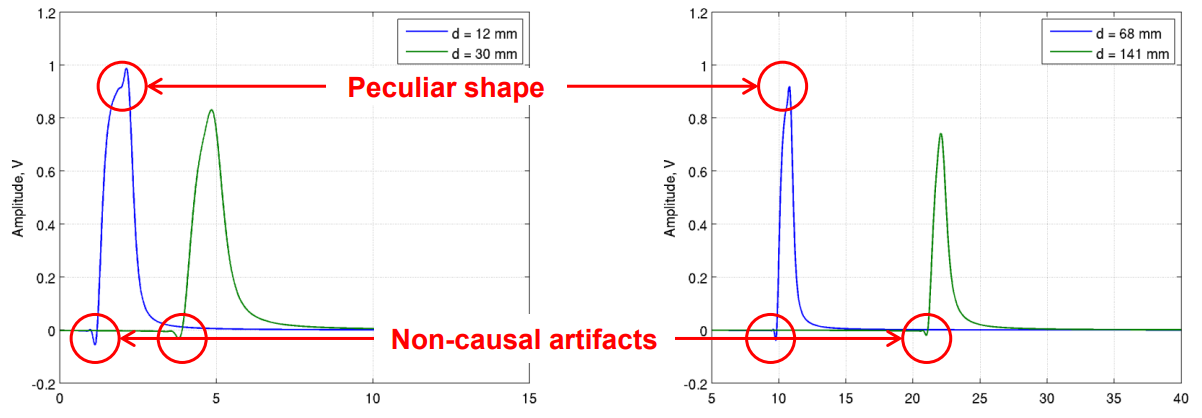This figure from the IEEE P802.3bj Task Force (2014) shows the effects of noncausal behavior in an interconnect.

## Transfer Functions for Causal LTI Systems

By definition, PCB signal behavior is causal as it obeys Maxwell’s equations. Incorrect models for signal behavior and dielectric behavior are what produce non-causal behavior. Similarly, incorrect mathematical descriptions of signal behavior, either in the time or frequency domain, will produce non-causal behavior in the time domain. Under a subsequent translation in time (i.e., as the signal travels to the observation point), the noncausal behavior that occurred before time t = 0 will produce some response in the system at time t > 0.

In a causal linear time invariant (LTI) system, we can easily model the behavior of the system in terms of transfer functions. This requires correctly describing the system in the frequency domain. Typical PCB simulation tools only consider steady-state behavior in the frequency domain for LTI systems; properly describing signal behavior in the frequency or time domain requires truncating the time-dependent signal source at t = 0 in these simulation tools. Is allows you to define the correct relationship between an interconnect’s transfer function, or a PDN’s transfer function, and the frequency content of a source signal.

### The System’s Transfer Function and Response

If you want to predict the system’s response in the time domain, then you need the system’s transfer function in the frequency domain, which must be known a priori. Going the other direction, if you want to determine the transfer function, you can do so using a measurement of the response and its Fourier transform. To begin, the source signal is defined in terms of a truncated impulse response h(t) at time t = 0 using the sign(x) function: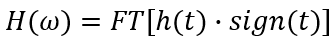Eq. (1): Causal transfer function in terms of the system’s impulse response function.

Note that the impulse response function h(t) defines the system response to a delta-function impulse at t = 0. Using the convolution theorem, one can define Kramers-Kronig relations between the real and imaginary parts of the transfer function: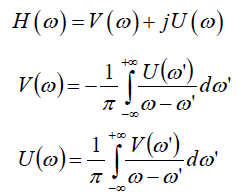Eq. (2): Kramers-Kronig relations for a causal transfer function.

This set of equations effectively tells you that if you know the impulse response for your system, which can be determined using Laplace analysis for the system’s governing differential equation, then you can determine the causal transfer function. Procedures for solving this coupled pair of integral equations are well-documented. Conversely, if you know the transfer function of the system, you can determine the causal impulse response function with an inverse Fourier transform. This can be reframed as a design problem in terms of measured S-parameters or transfer function for a real system. Once you know either of these, you can then calculate the causal impulse response function for the system. You can then determine signal behavior for any input signal using a convolution integral: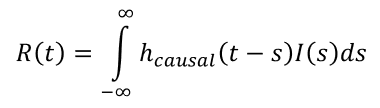Eq. (3): Time-domain system response in terms of the causal impulse response function.

where I(t) is the input signal in the time domain, and R(t) is the response in the time domain. Alternatively, you can calculate the input signal you must put into the system for a desired response function in the time-domain. Predicting the correct response in the time domain requires moving through the following process:

1. Determine the S-parameters or transfer function, either from measurements, numerical modeling (e.g., FDFD), or analytically using an accurate model. In PCBs, this requires accurate modeling of dispersion in PCB substrates; the wideband Debye model is widely regarded as the most accurate model for describing dispersion.
2. Calculate the impulse response function using the causal relationship with the transfer function shown above.
3. Use the causal impulse response function to calculate the time-domain response for your intended input signal, or calculate the input required to produce a desired output by using the convolution theorem in Eq. (3).

At NWES, we help innovators understand and model all aspects of their new designs, get them into production, and plan marketing campaigns to support product releases. If you're looking for a cutting-edge PCB design service bureau and thought leadership marketing services, contact NWES for a consultation.

* indicates required

## Our Clients and Partners